# PHP Explode

PHP explodes splits string by a given string and returns an array. It is one of the key PHP functions to perform string manipulations. It can be used for multiple purposes by exploding input string with a boundary string.

We have already seen PHP explode() and related functions in a previous tutorial. It replaces the deprecated PHP split() function.

This article will give a quick example and more variations on PHP explode. It will cover the basic ideas and usage practices of this built-in function.

The following quick example code uses PHP explode() function on a given string. It splits the string into pieces with the white space. The code outputs the exploded pieces in a form of an array.

## Quick Example

``````<?php
\$employees = "David Moshe Marco Steffan Devin William";
\$result = explode(" ", \$employees);

//Prints array
echo "Printing PHP array:";
print "<PRE>";
print_r(\$result);

// Iterates array generated using PHP explode
echo "Iterating exploded array:";
foreach (\$result as \$value) {
echo \$value . "<br/>";
}
?>
``````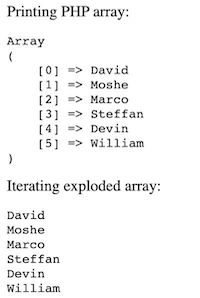## Usage syntax and parameters overview

The PHP explode() syntax is below followed by the parameter description. This is one of the heavily used string functions available in PHP.

``````explode(string \$boundaryString, string \$inputString, int \$limit = PHP_INT_MAX);
``````
• \$boundaryString – The separator about which the PHP explode will happen.
• \$inputString – The input string to be exploded.
• \$limit – Limit to the length of the output array returned by the explode() function. This is optional. It has a default value PHP_INT_MAX. It is the largest integer supported by the current PHP build. It also accepts a negative integer to set the limit.

This function returns an array of substrings exploded from the inputString.

When the \$bounsaryString is empty, then the explode() function will return value error.

Note: The PHP explode() function is binary-safe. It can process any string containing binary data also.

The function this is not binary safe ignores the rest once it finds any non-ASCII or null characters in a string. An example of a non-binary-safe function in PHP is strcoll().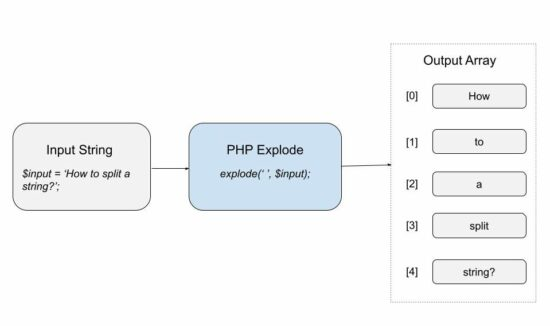## Variations on using PHP explode

The below example uses PHP explode() function by giving various input values. We will see the different behaviour of this function on receiving those inputs.

### Explode with empty separator

This example code passes an empty string as a separator to the PHP explode function. In this case, it returns a PHP value error.

``````
<?php
\$employees = "David Moshe Marco Steffan Devin William"
\$result = explode("", \$employees);
print "<PRE>";
print_r(\$result);
print "</PRE>";
?>
``````

It will return the following warning message on executing explode with empty separator.

``````Warning: explode(): Empty delimiter in <file> on line....
``````

### Explode string that is not having the boundary string

When the separator is not found in the given input string, the explode() will return the input string in an array.

``````<?php
\$employees = "David Moshe Marco Steffan Devin William";
\$result = explode(":", \$employees);
print "<PRE>";
print_r(\$result);
print "</PRE>";
?>
``````

The returned array will have the length 1. The single array element contains the whole input string as its value.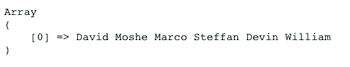Note: If \$limit contains a negative integer, it will return an empty array in this case.

### Explode with positive, negative limits

The \$limit parameter of the explode() function accepts negative values also. With a negative limit, the PHP explode output an array with length `substring_count - limit`.

``````<?php
\$string = 'Hello, World!, Learn, New';
print "<pre>";
print_r(explode(', ', \$string, 0));
print_r(explode(', ', \$string, 2));
print_r(explode(', ', \$string, 3));
print_r(explode(', ', \$string, - 1));
print_r(explode(', ', \$string, - 2));
?>
``````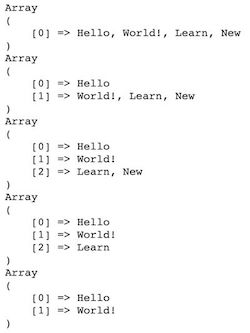## Related PHP functions like explode() to handle string splitting

This section shows examples of the related PHP functions to explode a string input. Those are,

1. preg_split()
2. str_split()
3. mb_split()
4. strtok()

The strtok() function explodes and tokenizes the input string.

### preg_split()

It splits a string by a regular expression. It finds the pattern on the string and breaks it into substrings. It accepts 4 parameters. The fourth parameter is the combination of the following.

1. PREG_SPLIT_NO_EMPTY – To returns only non-empty characters.
2. PREG_SPLIT_DELIM_CAPTURE – To capture the delimiter pattern to return.
3. PREG_SPLIT_OFFSET_CAPTURE – To capture the position of occurrence to return.

This example uses a regex pattern to split the input string. We have already seen examples for PHP string extract by regex.

examples/related-functions.php

``````<?php
\$string = ' Hello & World! & Welcome | to | learn new';
\$pattern = "/[\&,|]+/";
print "<pre>";
\$array = preg_split(\$pattern, \$string);
print_r(\$array);
print "</pre>";
?>
``````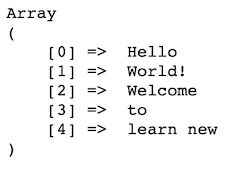### str_split()

This function splits the string into an array of characters. It accepts an optional \$length parameter to split the string into chunks.

examples/related-functions.php

``````<?php
\$string1 = 'Hello';
print "<pre>";
\$result = str_split(\$string1);
print_r(\$result);
print "</pre>";
?>
``````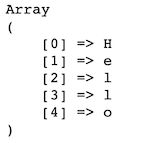### mb_split()

This function splits a multi-byte string by a regex pattern.

examples/related-functions.php

``````<?php
\$string2 = 'Welcome David!';
\$pattern = "\s";
print "<pre>";
\$result = mb_split(\$pattern, \$string2);
print_r(\$result);
print "</pre>";
?>
``````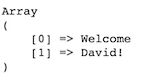### strtok()

This function tokenizes the input string with the given delimiter. It requires the input string for the first time only. In the subsequent calls, it continues tokenization by tracking the delimiter’s position.

``````<?php
\$string3 = "Owl-Parrot-Fox-Crane";
\$delimiter = "-";
\$token = strtok(\$string3, \$delimiter);

while (\$token !== false) {
echo \$token . "\n";
\$token = strtok(\$delimiter);
}
?>
``````

This code outputs the tokenized substring in each iteration. It removes the hyphen(-) from the input string and explodes it into tokens.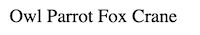## How to use PHP explode in web applications

PHP explode() is not only for handling strings. It is used in an effective way while creating web applications.

You may see some web forms asking to enter more data with a comma or space separation. In such cases, the PHP explode will help to parse the combo to break it into pieces.

For example, the form fields listed below accept more options.

• Languages known
• How do you know about us?

Let us see some of those examples below to use in a web application.

### Explode and load items into HTML dropdown

This code applies PHP explode to a comma-separated string. This input string contains country names. The explode will return an array of countries from the input string.

Then this code iterates the output array and loads the options to a HTML dropdown. Once we have seen how to load country-state dropdown from the database.

``````<?php
\$countries = "Germany,United Kingdom,France,Spain,United States";
\$result = explode(",", \$countries);
?>
<html>
<meta charset="utf-8">
<meta http-equiv="X-UA-Compatible" content="IE=edge">
<meta name="viewport" content="width=device-width, initial-scale=1">
<body>
<div class="container">
<div class="row">
<div class="label">Select Country</div>
<select class="input-select" name="country">
<option value="">Select</option>
<?php foreach(\$result as \$country){?>
<option value="<?php echo \$country;?>"><?php echo \$country;?></option>
<?php }?>
</select>
</div>
</div>
</body>
</html>
``````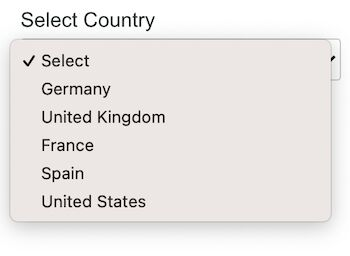This example generates a username automatically from the given email. It gets the email from the user via a HTML form.

It applies PHP explode on the entered email with respect to the separator ‘@’. The explode returns an array with length 2. The first element is taken as the username. The second element holds the domain name.

``````<?php
if (! empty(\$_POST["submit"])) {
if (! empty(\$_POST["email"])) {
\$string = \$_POST["email"];
\$result = explode("@", \$string, - 1);
}
}
?>
<html>
<meta charset="utf-8">
<meta http-equiv="X-UA-Compatible" content="IE=edge">
<meta name="viewport" content="width=device-width, initial-scale=1">
<body>
<div class="container">
<form action="" method="post">
<div class="row">
<div class="label">Enter Email</div>
<input type="text" name="email" class="input">
</div>
<div class="row">
<input type="submit" value="Submit" class="btn-submit"
name="submit">
</div>
<div class="row">
<?php
if (! empty(\$result)) {
echo "<strong>Username :</strong> " . \$result;
}
?>
</div>
</form>
</div>
</body>
</html>
``````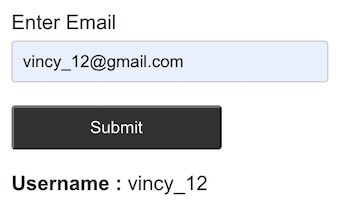### Explode user input languages known

This example code gets the comma-separated values from the user. The form field allows entering the languages known by the user.

On submit, the form posts the user data to the PHP. It explodes the posted data using PHP explode() function.

The output will be printed after exploding the data. This example prints the iterated language array elements below the form.

examples/get-languages-known.php

``````<?php
if (! empty(\$_POST["submit"])) {
if (! empty(\$_POST["languages"])) {
\$languages = \$_POST["languages"];
\$result = explode(",", \$languages);
}
}
?>
<html>
<title>PHP-Get languages known</title>
<meta charset="utf-8">
<meta http-equiv="X-UA-Compatible" content="IE=edge">
<meta name="viewport" content="width=device-width, initial-scale=1">
<body>
<div class="container">
<h4>Explode user input languages known</h4>
<form action="" method="post">
<div class="row">
<div class="label">Enter Languages <span class="note">(Enter comma-separated):</span></div>
<input type="text" name="languages" class="input">
</div>
<div class="row">
<input type="submit" value="Submit" class="btn-submit"
name="submit">
</div>
<div class="row">
<?php if(!empty(\$result)){?>
<div class="label font-bold">Languages known :</div>
<?php  foreach(\$result as \$language){?>
<div class="explode"> <?php echo \$language;?></div><?php } }?>
</div>
</form>
</div>
</body>
</html>
``````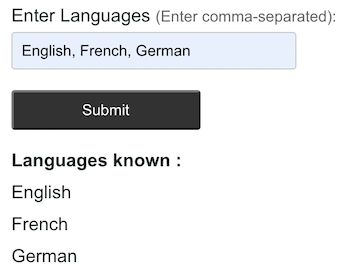## PHP explode example files

The below screenshot shows the file structure of the PHP explode example. On a landing page, it has the navigation to see the output of each explode example.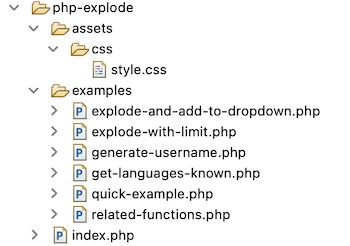## Conclusion

We have seen the basic idea on the PHP explode() function. Also, we saw the related PHP functions used for exploding strings.

All the above PHP explode examples will help to understand the usage practice. Also, it will guide you on how and where to use the PHP explode.